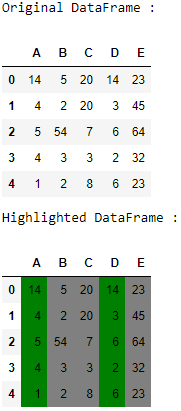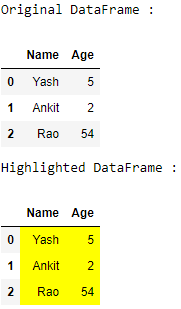Related Articles

# Highlight Pandas DataFrame’s specific columns using apply()

• Difficulty Level : Medium
• Last Updated : 17 Aug, 2020

Let us see how to highlight specific columns of a Pandas DataFrame. We can do this using the `apply()` function of the Styler class.

## Styler.apply()

Syntax : Styler.apply(func, axis = 0, subset = None, **kwargs)

Parameters :

• func : function should take a Series or DataFrame (depending on-axis), and return an object with the same shape. Must return a DataFrame with identical index and column labels when axis = None.
• axis : apply to each column (axis=0 or ‘index’) or to each row (axis=1 or ‘columns’) or to the entire DataFrame at once with axis = None
• subset : valid indexer to limit data to before applying the function.
• **kwargs : dict pass along to func.

Returns : Styler

Let’s understand with examples:

Example 1 :

 `# importing pandas as pd ``import` `pandas as pd `` ` `# creating the dataframe``df ``=` `pd.DataFrame({``"A"` `: [``14``, ``4``, ``5``, ``4``, ``1``],``                   ``"B"` `: [``5``, ``2``, ``54``, ``3``, ``2``],``                   ``"C"` `: [``20``, ``20``, ``7``, ``3``, ``8``], ``                   ``"D"` `: [``14``, ``3``, ``6``, ``2``, ``6``],``                   ``"E"` `: [``23``, ``45``, ``64``, ``32``, ``23``]}) `` ` `print``(``"Original DataFrame :"``)``display(df)`` ` `# function definition``def` `highlight_cols(x):``     ` `    ``# copy df to new - original data is not changed``    ``df ``=` `x.copy()``     ` `    ``# select all values to green color``    ``df.loc[:, :] ``=` `'background-color: green'``     ` `    ``# overwrite values grey color``    ``df[[``'B'``, ``'C'``, ``'E'``]] ``=` `'background-color: grey'``     ` `    ``# return color df``    ``return` `df `` ` `print``(``"Highlighted DataFrame :"``)``display(df.style.``apply``(highlight_cols, axis ``=` `None``))`

Output :Example 2 :

 `# importing pandas as pd ``import` `pandas as pd `` ` `# creating the dataframe``df ``=` `pd.DataFrame({``"Name"` `: [``"Yash"``, ``"Ankit"``, ``"Rao"``],``                   ``"Age"` `: [``5``, ``2``, ``54``]}) `` ` `print``(``"Original DataFrame :"``)``display(df)`` ` `# function definition``def` `highlight_cols(x):``     ` `    ``# copy df to new - original data is not changed``    ``df ``=` `x.copy()``     ` `    ``# select all values to yellow color``    ``df.loc[:, :] ``=` `'background-color: yellow'``     ` `    ``# return color df``    ``return` `df `` ` `print``(``"Highlighted DataFrame :"``)``display(df.style.``apply``(highlight_cols, axis ``=` `None``))`

Output :Attention geek! Strengthen your foundations with the Python Programming Foundation Course and learn the basics.

To begin with, your interview preparations Enhance your Data Structures concepts with the Python DS Course. And to begin with your Machine Learning Journey, join the Machine Learning – Basic Level Course

My Personal Notes arrow_drop_up